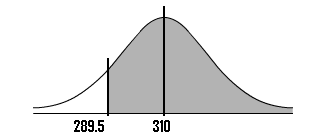# Normal Approximation to the Binomial

The normal approximation to the binomial is when you use a continuous distribution (the normal distribution) to approximate a discrete distribution (the binomial distribution). According to the Central Limit Theorem, the sampling distribution of the sample means becomes approximately normal if the sample size is large enough.

## Normal Approximation to the Binomial: n * p and n * q Explained

Need help with a homework question? Check out our tutoring page!
The first step into using the normal approximation to the binomial is making sure you have a “large enough sample”. How large is “large enough”? You figure this out with two calculations: n * p and n * q .

• n is your sample size,
• p is your given probability.
• q is just 1 – p. For example, let’s say your probability p is .6. You would find q by subtracting this probability from 1: q = 1 – .6 = .4. Percentages (instead of decimals) can make this a little more understandable; if you have a 60% chance of it raining (p) then there’s a 40% probability it won’t rain (q).

When n * p and n * q are greater than 5, you can use the normal approximation to the binomial to solve a problem.

## Normal Approximation: Example #1 (Video)

Watch the video for an example:

## Normal Approximation: Example #2

Sixty two percent of 12th graders attend school in a particular urban school district. If a sample of 500 12th grade children are selected, find the probability that at least 290 are actually enrolled in school.

## Part 1: Making the Calculations

Step 1: Find p,q, and n:

• The probability p is given in the question as 62%, or 0.62
• To find q, subtract p from 1: 1 – 0.62 = 0.38
• The sample size n is given in the question as 500

Step 2: Figure out if you can use the normal approximation to the binomial. If n * p and n * q are greater than 5, then you can use the approximation:
n * p = 310 and n * q = 190.
These are both larger than 5, so you can use the normal approximation to the binomial for this question.

Step 3: Find the mean, μ by multiplying n and p:
n * p = 310
(You actually figured that out in Step 2!).

Step 4: Multiply step 3 by q :
310 * 0.38 = 117.8.

Step 5: Take the square root of step 4 to get the standard deviation, σ:
√(117.8)=10.85
Note: The formula for the standard deviation for a binomial is √(n*p*q).

## Part 2: Using the Continuity Correction Factor

Step 6: Write the problem using correct notation. The question stated that we need to “find the probability that at least 290 are actually enrolled in school”. So:
P(X ≥ 290)

Step 7: Rewrite the problem using the continuity correction factor:
P (X ≥ 290-0.5) = P (X ≥ 289.5)
Note: The CCF table is listed in the above image, but if you haven’t used it before, you may want to view the video in the continuity correction factor article.

Step 8: Draw a diagram with the mean in the center. Shade the area that corresponds to the probability you are looking for. We’re looking for X ≥ 289.5, so:Step 9: Find the z-score.
You can find this by subtracting the mean (μ) from the probability you found in step 7, then dividing by the standard deviation (σ):
(289.5 – 310) / 10.85 = -1.89

Step 10: Look up the z-value in the z-table:
The area for -1.89 is 0.4706.
0.4706 + 0.5 = 0.9706.

That’s it! The probability is .9706, or 97.06%.

Check out our YouTube channel for hundreds more statistics help videos!

## References

Kotz, S.; et al., eds. (2006), Encyclopedia of Statistical Sciences, Wiley.
Everitt, B. S.; Skrondal, A. (2010), The Cambridge Dictionary of Statistics, Cambridge University Press.
Vogt, W.P. (2005). Dictionary of Statistics & Methodology: A Nontechnical Guide for the Social Sciences. SAGE.
Lindstrom, D. (2010). Schaum’s Easy Outline of Statistics, Second Edition (Schaum’s Easy Outlines) 2nd Edition. McGraw-Hill Education

CITE THIS AS:
Stephanie Glen. "Normal Approximation to the Binomial" From StatisticsHowTo.com: Elementary Statistics for the rest of us! https://www.statisticshowto.com/probability-and-statistics/binomial-theorem/normal-approximation-to-the-binomial/